# Urinary Excretion Data (Disposition Viewed from Urine only)

| Home | | Biopharmaceutics and Pharmacokinetics |

## Chapter: Biopharmaceutics and Pharmacokinetics : Compartment Modelling

In the absence of plasma level-time data, useful information can still be obtained from urine data regarding elimination kinetics of a drug.

URINARY EXCRETION DATA

(Disposition Viewed from Urine only)

In the absence of plasma level-time data, useful information can still be obtained from urine data regarding elimination kinetics of a drug. The method has several advantages in the analysis of a pharmacokinetic system:

1. The method is useful when there is lack of sufficiently sensitive analytical techniques to measure concentration of drugs in plasma with accuracy.

2. The method is non-invasive and therefore better subject compliance is assured.

3. The method is more convenient since it involves collection of urine samples in comparison to drawing of blood periodically.

4. A less sensitive analytical method is required for determining urine drug concentration as compared to plasma concentrations. If urine drug concentrations are low, assaying of larger sample volumes is relatively easy.

5. First-order elimination, excretion and absorption rate constants and fraction excreted unchanged can be computed from such data. First-order metabolism or extra-renal excretion rate constant can also be calculated subsequently from the difference (KE – Ke) = Km.

6. Direct measurement of bioavailability, both absolute and relative, is possible without the necessity of fitting the data to a mathematical model.

7. When coupled with plasma level-time data, it can also be used to estimate renal clearance of unchanged drug according to following equation: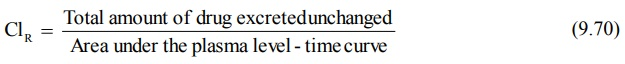If Vd is known, total systemic clearance and nonrenal clearance can also be calculated.

One cannot, however, compute Vd and ClT from urine data alone. One must also remember that urinary excretion data is not an accurate substitute for the plasma level data. At best, the data can be employed as a rough estimate of the pharmacokinetic parameters. Moreover, if the drug product provides a very slow drug release or if the drug has a very long biological half-life, the resulting low urinary drug concentration may be too dilute to be assessed with accuracy. In the latter case, i.e. for drugs with long t½, urine may have to be collected for several days to account for total drug excreted.

### Criteria for Obtaining Valid Urinary Excretion Data

1.        A significant amount of drug must be excreted unchanged in the urine (at least 10%).

2.        The analytical method must be specific for the unchanged drug; metabolites should not interfere.

3.        Water-loading should be done by taking 400 ml of water after fasting overnight, to promote diuresis and enable collection of sufficient urine samples.

4.        Before administration of drug, the bladder must be emptied completely after 1 hour from water-loading and the urine sample taken as blank. The drug should then be administered with 200 ml of water and should be followed by 200 ml given at hourly intervals for the next 4 hours.

5.        Volunteers must be instructed to completely empty their bladder while collecting urine samples.

6.        Frequent sampling should be done in order to obtain a good curve.

7.        During sampling, the exact time and volume of urine excreted should be noted.

8.        An individual collection period should not exceed one biological half-life of the drug and ideally should be considerably less.

9.        Urine samples must be collected for at least 7 biological half-lives in order to ensure collection of more than 99% of excreted drug.

10.   Changes in urine pH and urine volume may alter the urinary excretion rate.

TABLE 9.5

Urinary Excretion Data following i.v. Bolus of 100 mg of a DrugThe urine data can be set as shown in the Table 9.5. Observations include times of urine collection, volumes collected and concentration of unchanged drug in each sample. These data are treated to derive further information.

### Determination of KE from Urinary Excretion Data

The first-order elimination (and excretion) rate constants can be computed from urine data by two methods:

1. Rate of excretion method, and

2. Sigma-minus method.

Rate of Excretion Method: The rate of urinary drug excretion dXu/dt is proportional to the amount of drug in the body X and written as:where Ke = first-order urinary excretion rate constant. According to first-order disposition kinetics, X = Xo e–KEt (equation 9.5). Substituting it in above equation yields: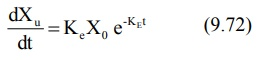where Xo = dose administered (i.v. bolus). Transforming to log form the equation becomes: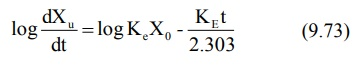The above equation states that a semilog plot of rate of excretion versus time yields a straight line with slope –KE/2.303 (Fig. 9.10). It must therefore be remembered that the slope of such an excretion rate versus time plot is related to elimination rate constant KE and not to excretion rate constant Ke. The excretion rate constant can be obtained from the Y-intercept (log Ke Xo). Elimination half-life and nonrenal elimination rate constant can then be computed from KE and Ke.

An advantage of excretion rate method is that for drugs having long half-lives, urine may be collected for only 3 to 4 half-lives. Moreover, there is no need to collect all urine samples since collection of any two consecutive urine samples yield points on the rate plot from which a straight line can be constructed.Fig. 9.10 Semilog plot of excretion rate versus mid-point time of urine collection period for computing elimination rate constant after i.v. bolus administration.

Sigma-Minus Method: A disadvantage of rate of excretion method in estimating KE is that fluctuations in the rate of drug elimination are observed to a high degree and in most instances, the data are so scattered that an estimate of half-life is difficult. These problems can be minimized by using the alternative approach called as sigma-minus method.

From an earlier equation:Integration of equation 9.72 yields: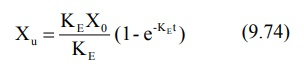where Xu = cumulative amount of drug excreted unchanged in urine at any time t. As time approaches infinity i.e. after 6 to 7 half-lives, the value e–KE becomes zero and therefore the cumulative amount excreted at infinite time Xu can be given by equation: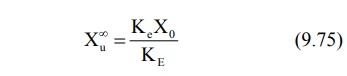Substitution of equation 9.75 in equation 9.74 and rearrangement yields: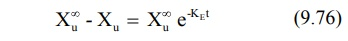Converting to logarithms, we get: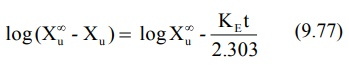where (Xu – Xu) = amount remaining to be excreted i.e. ARE at any given time. A semilog plot of ARE versus t yields a straight line with slope -KE/2.303. The method is,

therefore, also called as ARE plot method. A disadvantage of this method is that total urine collection has to be carried out until no unchanged drug can be detected in the urine i.e. upto 7 half-lives, which may be tedious for drugs having long t½.

The equations until now for computing KE from the urinary excretion data apply to a drug that fits one-compartment model and given as i.v. bolus. Similarly, data obtained during constant rate i.v. infusion can be used to evaluate the elimination rate constant. The equation that describes the urinary excretion rate of unchanged drug when administered as i.v. bolus also applies when it is administered as i.v. infusion. Thus:For a drug given as i.v. infusion, the amount of drug in the body X is given by equation (described earlier):Substitution of equation 9.34 in equation 9.71 and integration of the same yields:When the drug has been infused for a period long enough to attain steady-state in the plasma, the term e–KEt approaches zero and the above equation reduces to:A regular plot of cumulative amount of drug excreted Xu versus t yields a curvilinear plot the linear portion of which has a slope KeRo/KE. Extrapolation of linear segment to time axis yields x-intercept equal to 1/KE since when X u = 0, t= 1/KE (Fig. 9.11).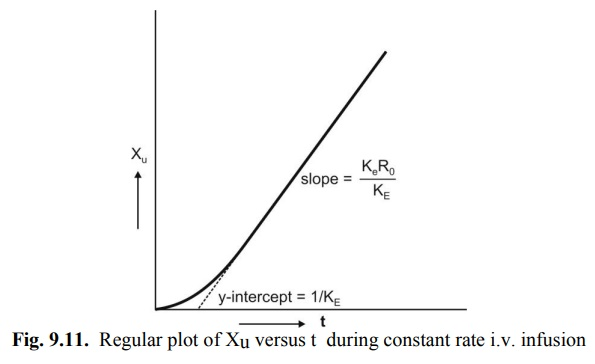Fig. 9.11. Regular plot of Xu versus t during constant rate i.v. infusion

Relationships for rate of excretion when the drug is administered e.v. can also be given similarly. Thus: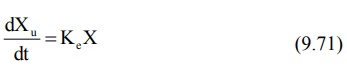For a drug given e.v. and absorbed by a first-order process, X is given as: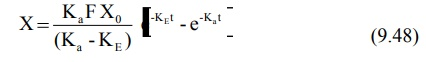Substitution of equation 9.48 in equation 9.71 and integration of the same yields:At time infinity, the equation 9.80 reduces to:Substitution of equation 9.81 in equation 9.80 and subsequent rearrangement yields: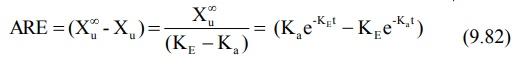A semilog plot of (Xu – Xu) versus t results in a biexponential curve and if Ka > KE, the slope of the terminal linear portion of the curve will define KE of the drug. The absorption rate constant Ka can be estimated by the method of residuals using the same data i.e. equation 9.82.

Urinary excretion data after oral administration can also be treated according to Wagner-Nelson method to calculate Ka by construction of % ARA plots. The method requires urine collection for sufficiently long time to ensure accurate estimation of KE but need not be collected to time infinity. The equation derived to relate % ARA with urinary excretion rate is:A semilog plot of % ARA versus t yields a straight line with slope –Ka/2.303.

Accurate determination of Ka from urinary excretion data is possible only for drugs with slow rate of absorption since for drugs with rapid absorption, collection of urine samples at very short intervals of time is difficult.